# CHOOSECOLS function in Excel to get columns from array or range

This tutorial will introduce you to a new Excel 365 dynamic array function named CHOOSECOLS and show how you can use it to extract any specific columns from an array.

Imagine that you are working with a dataset of hundreds or thousands of columns. Obviously, some columns are more important than others, and naturally you may want to read their data first. Excel 365 offers a perfect function for the job, which can instantly retrieve specific certain from an array, so you can focus on the most relevant information.

## Excel CHOOSECOLS function

The CHOOSECOLS function in Excel is designed to return the specified columns from an array or range.

The syntax includes the following arguments:

CHOOSECOLS(array, col_num1, [col_num2], …)

Where:

Array (required) - the source array or range.

Col_num1 (required) - an integer specifying the first column to return.

Col_num2, … (optional) - index numbers of additional columns to return.

And this is how the CHOOSECOLS function may look like in your Excel: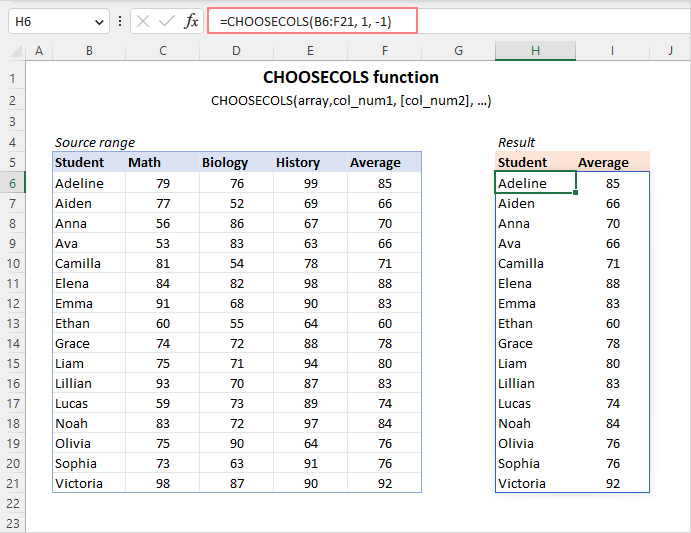### CHOOSECOLS function availability

Currently, the CHOOSECOLS function is available in Excel for Microsoft 365 (Windows and Mac) and Excel for the web.

Tip. To extract some rows from a range or array, the CHOOSEROWS function may come in handy.

## How to use CHOOSECOLS function in Excel

CHOOSECOLS is a dynamic array function, so it handles arrays natively. The formula is to be entered in just one cell - the upper-left cell of the destination range - and it automatically spills into as many columns as specified it its arguments and as many rows as there are in the original array. The result is a single dynamic array, which is called a spill range.

To make a CHOOSECOLS formula in Excel, this is what you need to do:

1. For array, supply a range of cells or an array of values.
2. For col_num, provide a positive or negative integer indicating which column to return. A positive number pulls a corresponding column from the left side of the array, a negative number - from the right side of the array. To get multiple columns, you can define their numbers in separate arguments or in one argument in the form of an array constant.

For example, to get columns 2, 3 and 4 from the range A4:E19, the formula is:

`=CHOOSECOLS(A4:E19, 2, 3, 4)`

Alternatively, you can use a horizontal array constant such as {2,3,4} or a vertical array constant such as {2;3;4} to specify the column numbers:

`=CHOOSECOLS(A4:E19, {2,3,4})`

`=CHOOSECOLS(A4:E19, {2;3;4})`

All three formulas above will deliver the same result: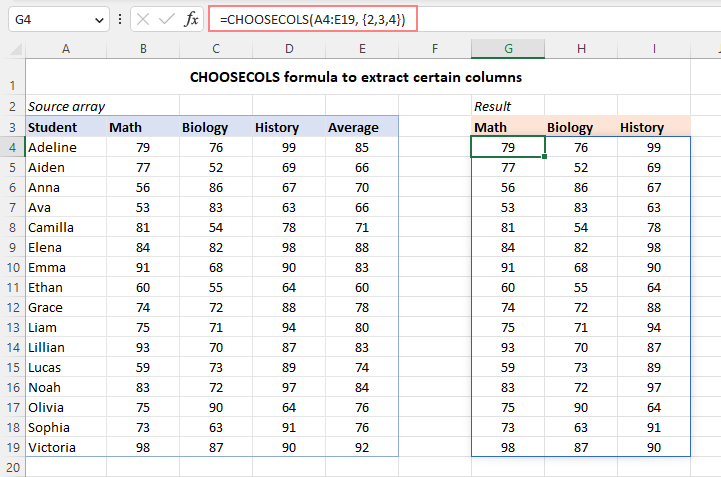In some situations, you may find it more convenient to input the column numbers in some cells, and then reference those cells individually or provide a single range reference. For example:

`=CHOOSECOLS(A4:E19, G4, H4, I4)`

`=CHOOSECOLS(A4:E19, G4:I4)`

This approach gives you more flexibility - to extract any other columns, you simply type different numbers in the predefined cells without having to modify the formula itself.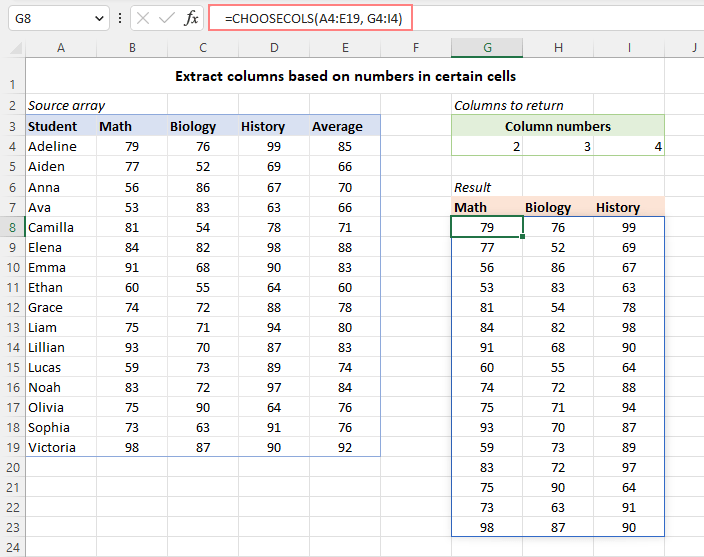Now that you know the essentials, let's dive into the extras and explore a slightly more complex CHOOSECOLS formulas to handle specific scenarios.

## Get last columns from range

To return one or more columns from the end of a range, supply negative numbers for the col_num arguments. This will make the function start counting columns from the right side of the array.

For instance, to get the last column from the range A4:D13, use this formula:

`=CHOOSECOLS(A4:E19, -1)`

To extract the last two columns, use this one:

`=CHOOSECOLS(A4:E19, -2, -1)`

To return the last 2 columns in reverse order, change the order of the col_num arguments like this:

`=CHOOSECOLS(A4:E19, -1, -2)`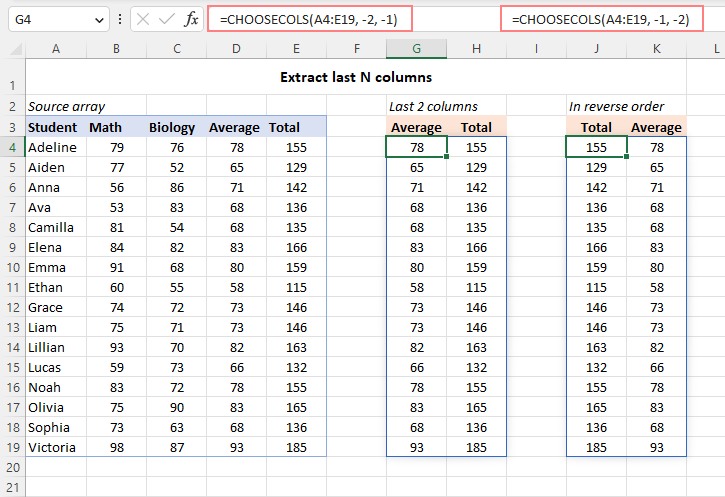## Get every other column in Excel

To extract every other column from a given range, you can use CHOOSECOLS together with several other functions. Below there are two versions of the formula for extracting odd and even columns.

To get odd columns (such as 1, 3, 5, etc.), the formula is:

`=CHOOSECOLS(A4:E19, SEQUENCE(ROUNDUP(COLUMNS(A4:E19)/2, 0), 1, 1, 2))`

To return even columns (such as 2, 4, 6, etc.), the formula takes this form:

`=CHOOSECOLS(A4:E19, SEQUENCE(ROUNDDOWN(COLUMNS(A4:E19)/2, 0), 1, 2, 2))`

The screenshot below shows the first formula in action: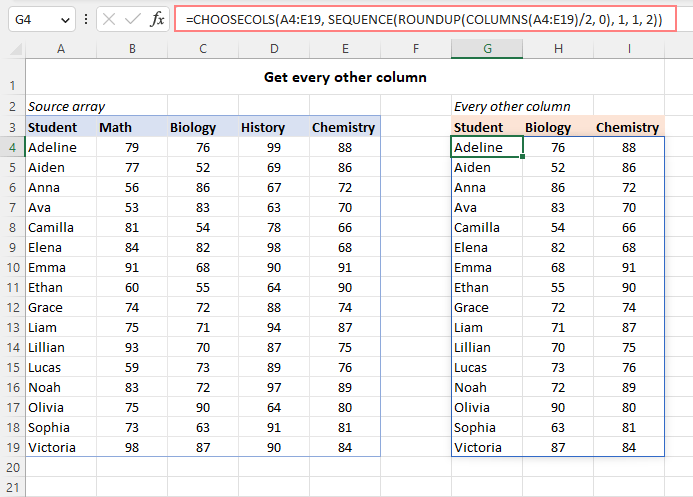How this formula works:

Brief explanation: The CHOOSECOLS function returns every other column based on an array of sequential odd or even numbers produced by the SEQUENCE function.

A detailed formula break-down:

The first step is to calculate how many columns to return. For this, we use one of these formulas:

`ROUNDUP(COLUMNS(A4:E19)/2, 0)`

or

`ROUNDDOWN(COLUMNS(A4:E19)/2, 0)`

COLUMNS counts the total number of columns in the source range. You divide that number by 2, and then, depending on whether you are extracting odd or even columns, round the quotient either upward or downward to the integer with the help of ROUNDUP or ROUNDDOWN. Rounding is needed in case the source range contains an odd number of columns, which leaves a remainder when divided by 2.

Our source range has 5 columns. So, for odd columns ROUNDUP(5/2, 0) returns 3, while for even columns ROUNDDOWN(5/2, 0) returns 2.

The returned number is served to the first argument (rows) of the SEQUENCE function.

For odd columns, we get:

`SEQUENCE(3, 1, 1, 2)`

This SEQUENCE formula generates an array of numbers consisting of 3 rows and 1 column, starting at 1 and incremented by 2, which is {1;3;5}.

For even columns, we have:

`SEQUENCE(2, 1, 2, 2)`

In this case, SEQUENCE produces an array of numbers consisting of 2 rows and 1 column, starting at 2 and incremented by 2, which is {2;4}.

The above array goes to the col_num1 argument of CHOOSECOLS, and you get the desired result.

## Flip an array horizontally in Excel

To reverse the order of columns in an array from left to right, you can use the CHOOSECOLS, SEQUENCE and COLUMNS functions together in this way:

`=CHOOSECOLS(A4:D19, SEQUENCE(COLUMNS(A4:D19)) *-1)`

As a result, the original range is flipped horizontally like shown in the image below: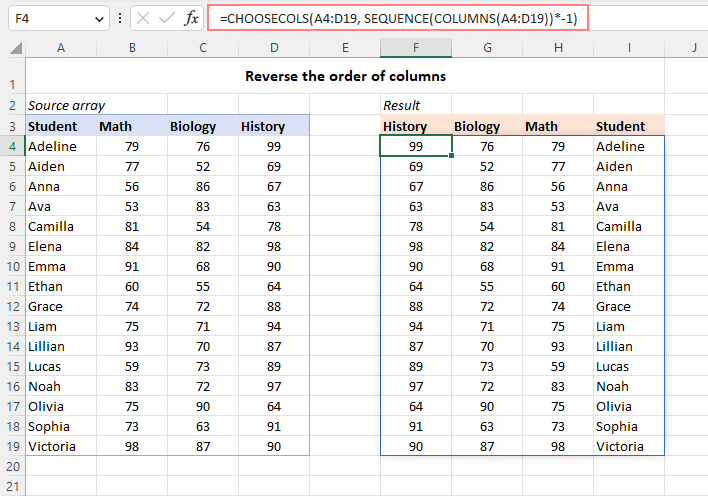How this formula works:

Here, we use the SEQUENCE function to generate an array containing as many sequential numbers as there are columns in the source array. For this, we nest COLUMNS(A4:D13) in the rows argument:

`SEQUENCE(COLUMNS(A4:D19))`

The other arguments (columns, start, step) are omitted, so they default to 1. As a result, SEQUENCE produces an array of sequential numbers such as 1, 2, 3, …, n, where n is the index of the last column in the array. To force the CHOOSECOLS function to count the columns from right to left, we multiply each element of the generated sequence by -1. As a result, we get an array of negative numbers such as {-1;-2;-3}, which goes to the col_num argument of CHOOSECOLS, instructing it to return the corresponding columns from the right side of the array:

`CHOOSECOLS(A4:D19, {-1;-2;-3;-4})`

## Extract columns based on string with numbers

In situation when the index numbers of the target columns are provided in the form of a text string, you can use the TEXTSPLIT function to split the string by a given delimiter, and then pass the resulting array of numbers to CHOOSECOLS.

Let's say the column numbers are listed in cell H3, separated by a comma and a space. To get the columns of interest, use this formula:

`=CHOOSECOLS(A4:E19, TEXTSPLIT(H3, ", ") *1)`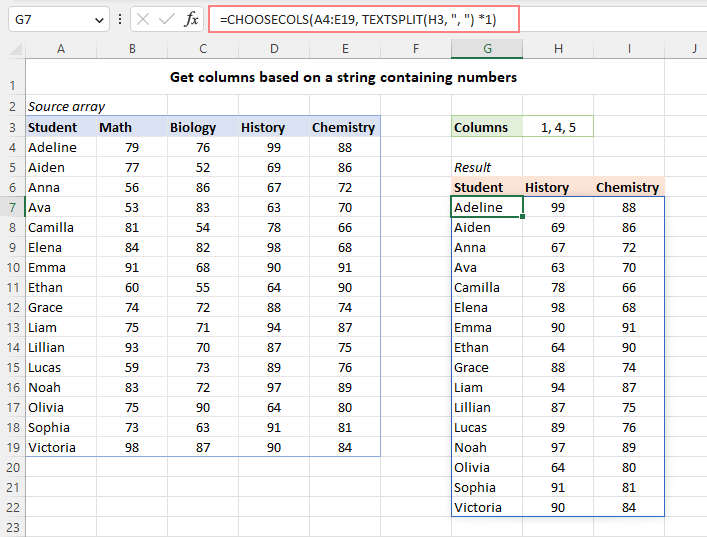How this formula works:

First, you split a string by a given delimiter (a comma and a space in our case):

`TEXTSPLIT(H3, ", ")`

An intermediate result is an array of text values such as {"1","4","5"}. To convert text to numbers, multiply the array items by 1 or perform any other math operation that does not change the original values.

`TEXTSPLIT(H3, ", ") *1`

This produces an array of numeric values {1,4,5} that the CHOOSECOLS function can process, and you'll get the result you are looking for:

`CHOOSECOLS(A4:E19, {1,4,5})`

## Extract columns from multiple ranges

To get particular columns from several non-contiguous ranges, you first merge all the ranges into one with the help of the VSTACK function, and then handle the merged range with CHOOSECOLS.

For example, to return columns 1 and 3 from the ranges A4:D8, A12:D15 and A19:D21, the formula is:

`=CHOOSECOLS(VSTACK(A4:D8, A12:D15, A19:D21), 1, 3)`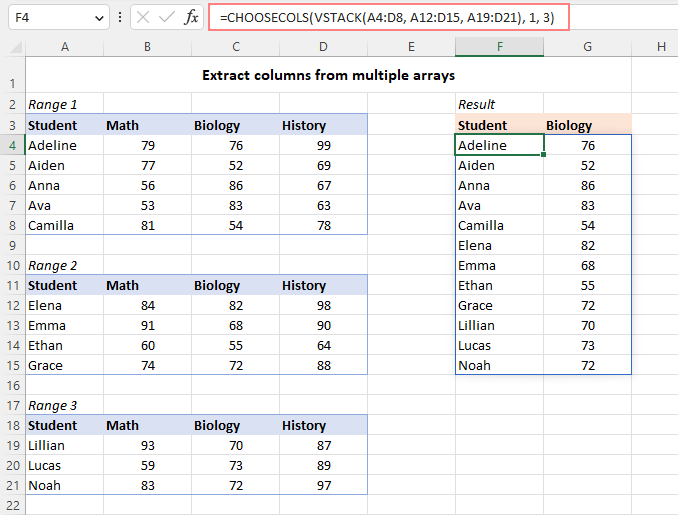## CHOOSECOLS function not working

If the CHOOSECOLS formula throws an error, it's most likely to be one of the following.

### #VALUE! error

Occurs if the absolute value of any col_num argument is zero or greater than the total number of columns in the referred array.

### #NAME? error

Occurs if the function's name is misspelled or the function is not available in your Excel version. Currently, CHOOSECOLS is only supported in Excel 365 and Excel for the web. For more details, see How to fix #NAME error in Excel.

### #SPILL! error

Occurs when something prevents the formula from spilling the results into neighboring cells. To fix it, just clear the obstructing cells. For more information, please see How to fix #SPILL! error in Excel.

That's how to use the CHOOSECOLS function in Excel to return particular columns from a range or array. Thank you for reading and see you on our blog next week!

Excel CHOOSECOLS formula - examples (.xlsx file)